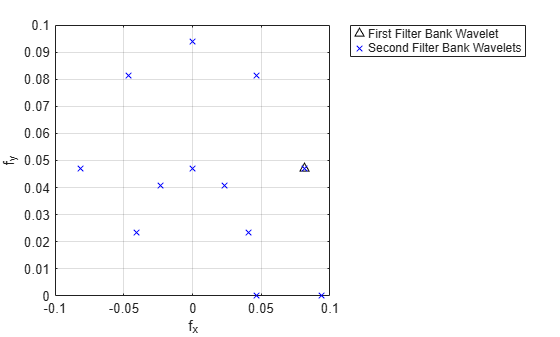paths

Scattering paths

Description

spaths = paths(sf) returns the scattering paths for all orders of the scattering network, sf. spaths is a cell array of MATLAB® tables with n elements, where n is the number of orders in the scattering network.

example

[spaths,npaths] = paths(sf) returns the number of paths in each order as n-by-1 column vector, where n is the number of orders in the scattering network. The sum of the elements of npaths is the total number of scattering paths.

Examples

collapse all

Create an image scattering network with an image size of 256-by-256 and invariance scale equal to the minimum of the image size. The default OptimizePath value is 1 (true).

sf = waveletScattering2('ImageSize',[256 256],'InvarianceScale',128)
sf =
waveletScattering2 with properties:

ImageSize: [256 256]
InvarianceScale: 128
NumRotations: [6 6]
QualityFactors: [1 1]
Precision: "single"
OversamplingFactor: 0
OptimizePath: 1

Obtain the number of scattering paths in each order. Display the total number of scattering paths.

[spaths,npaths] = paths(sf);
sum(npaths)
ans = 391

Set the OptimizePath value of the network to false. Display the total number of scattering paths. For the modified network, the scattering transform does not reduce the number of paths to compute based on a bandwidth consideration.

sf.OptimizePath = false;
[spaths,npaths] = paths(sf);
sum(npaths)
ans = 571

This example shows how the OptimizePath property can affect the scattering paths that include a specific wavelet.

Create the default wavelet image scattering network. Obtain all the wavelet filters and center spatial frequencies for the network. Obtain all the scattering paths. Display the total number of paths.

sf = waveletScattering2
[~,psifilters,f] = filterbank(sf);
[spaths,npaths] = paths(sf);
disp(['Total Number of Paths: ',num2str(sum(npaths))])
sf =

waveletScattering2 with properties:

ImageSize: [128 128]
InvarianceScale: 64
NumRotations: [6 6]
QualityFactors: [1 1]
Precision: 'single'
OversamplingFactor: 0
OptimizePath: 1

Total Number of Paths: 241

Display the number of wavelet filters in each filter bank.

disp(['Filter Bank 1: ',num2str(size(psifilters{1},3))]);
disp(['Filter Bank 2: ',num2str(size(psifilters{2},3))]);
Filter Bank 1: 24
Filter Bank 2: 24

Choose a wavelet from the first filter bank and display its spatial center frequency. Use spaths to find all the three-element paths that include the chosen wavelet. Display the paths.

waveletA = 14;
disp(['Center Frequency: ',num2str(f{1}(waveletA,:))]);
ind = find(spaths{3}.path(:,2)==waveletA);
spaths{3}(ind,:)
Center Frequency: 0.08119    0.046875

ans =

6x1 table

path
_____________

0    14    19
0    14    20
0    14    21
0    14    22
0    14    23
0    14    24

Plot the center frequencies of the wavelet filters on the paths.

plot(f{1}(waveletA,1),f{1}(waveletA,2),'k^');
xlabel('f_x')
ylabel('f_y')
hold on
waveletBs = spaths{3}.path(ind,3);
plot(f{2}(waveletBs,1),f{2}(waveletBs,2),'bx');
grid on
legend('First Filter Bank Wavelet','Second Filter Bank Wavelets',...
'Location','northeastoutside')Now set the OptimizePath property of the scattering network sf to false. Obtain the wavelet filters, center spatial frequencies, and scattering paths of the network.

sf.OptimizePath = false
[~,psifilters2,f2] = filterbank(sf);
[spaths2,npaths2] = paths(sf);
disp(['Total Number of Paths: ',num2str(sum(npaths2))])
sf =

waveletScattering2 with properties:

ImageSize: [128 128]
InvarianceScale: 64
NumRotations: [6 6]
QualityFactors: [1 1]
Precision: 'single'
OversamplingFactor: 0
OptimizePath: 0

Total Number of Paths: 385

Choose the same wavelet as above. To confirm it is the same wavelet, display its spatial center frequency. Use spaths to find all the three-element paths that include the wavelet. Because OptimizePath is set to false, the wavelet filter has more children.

waveletA = 14;
disp(['Center Frequency: ',num2str(f2{1}(waveletA,:))]);
ind = find(spaths2{3}.path(:,2)==waveletA);
spaths2{3}(ind,:)
Center Frequency: 0.08119    0.046875

ans =

12x1 table

path
_____________

0    14    13
0    14    14
0    14    15
0    14    16
0    14    17
0    14    18
0    14    19
0    14    20
0    14    21
0    14    22
0    14    23
0    14    24

Plot the center frequencies of the wavelet filters on the paths. Some of child filters have center frequencies higher than the chosen wavelet.

figure
plot(f2{1}(waveletA,1),f2{1}(waveletA,2),'k^');
xlabel('f_x')
ylabel('f_y')
hold on
waveletBs = spaths2{3}.path(ind,3);
plot(f2{2}(waveletBs,1),f2{2}(waveletBs,2),'bx');
grid on
legend('First Filter Bank Wavelet','Second Filter Bank Wavelets',...
'Location','northeastoutside')Input Arguments

collapse all

Wavelet image scattering network, specified as a waveletScattering2 object.

Output Arguments

collapse all

Scattering paths of all orders of the scattering network, returned as a cell array of MATLAB tables. spaths has n elements, where n is the number of orders in the scattering network.

Each MATLAB table in spaths contains a single variable, path. The variable path is a row vector with one column for each element of the path. The scalar 0 denotes the original image. Positive integers in the Lth column denote the corresponding wavelet filter in the (L−1)th filter bank. Wavelet bandpass filters are ordered by decreasing center frequency. There are NumRotations wavelets per center frequency pair.

Number of scattering paths in each order of the scattering network. npaths is a no-by-1 column vector where no is the number of orders in the scattering network. The sum of the elements of npaths is the total number of scattering paths.